# Major Concepts Of Ancient Egyptian Mathematics

###### ByRamraj Saini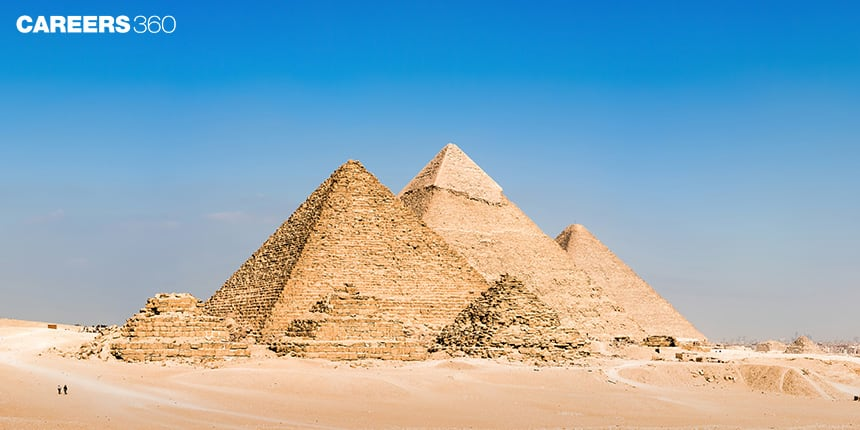###### Synopsis

Mathematics can be rooted in different ancient civilizations. One of the important civilizations is Egypt which contributed heavily to the development of Mathematics. Read this article to know more about it.###### Synopsis

Mathematics can be rooted in different ancient civilizations. One of the important civilizations is Egypt which contributed heavily to the development of Mathematics. Read this article to know more about it.

Like the many fascinating things the Egyptian civilisation gave to the world, ancient Mathematics is another important contribution by them. Ancient Egyptians contributed heavily to the development of Mathematics and Accounting. In pre-modern Mathematics, major fields were Algebra and Geometry. It was studied in various forms in different ancient civilizations like Egypt, India, Greece and China.

There were continuous floods in Egypt due to the Nile river and the floods wiped out the boundaries of fields of different owners. These boundaries had to be redrawn and therefore they developed different geometric techniques to calculate the area for land management. These methods were also used for land measurement, construction of Pyramids, temples, irrigation systems, storage facilities, and basic accounting. In architectural engineering determination and calculation of the area and volume of three-dimensional shapes like pyramids is required. The Egyptians devised some sophisticated techniques for the application of Mathematics in everyday life.

The Ancient Egyptians had very accurate representations of numbers, decimal systems, and fractions. They used a ten-digit system to determine the volume of a squat circle or an oxen-shaped pyramid. Similarly, they also knew the fact that the height of the frustum of a square pyramid was equal to one-third of its circumference.

## Major Mathematics Concepts In Ancient Egypt

Egyptians are largely responsible for the development of Mathematics. They used similar formulas and representation systems as we use today. Their techniques and knowledge were passed on to the Greeks. The Egyptian civilisation built many great monuments and this shows that they had a good knowledge of applied Mathematics, based on developing good techniques for surveying and building. Following are some major developments in Mathematics.

## Numerals

In ancient Egypt, the representation of the number system was given as a base of ten. Number one was depicted as a single stock, for the depiction of two, and three… they used single stock, two stock, and three stock…respectively. The Egyptian number system was additive. Large numbers can be represented by the collection of glyphs. The value of that number can be calculated by simple addition. The following image clearly shows the representation of the number system in ancient Egypt.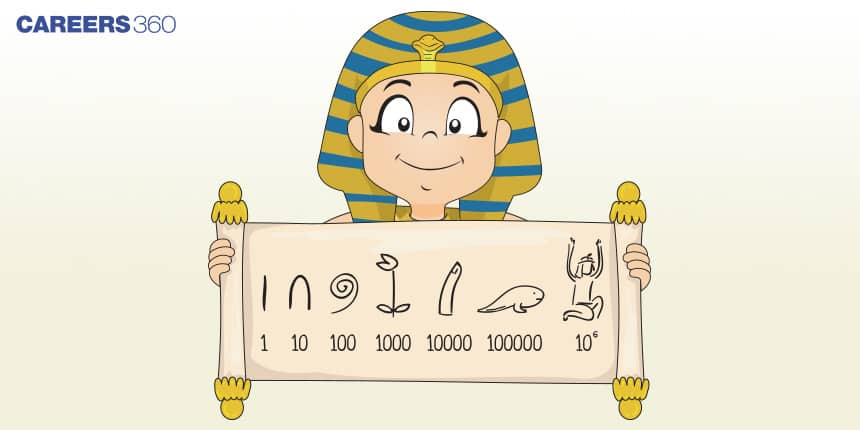Fractions

The Egyptians exclusively used fractions in the form of 1/n. A glyph represents the fraction ½ as a folded rope in two lines. The fraction ⅔ is represented as a mouth with superimposed two strokes. The following image clearly shows the representation of fractions.

### Hieroglyphics For Some Fractions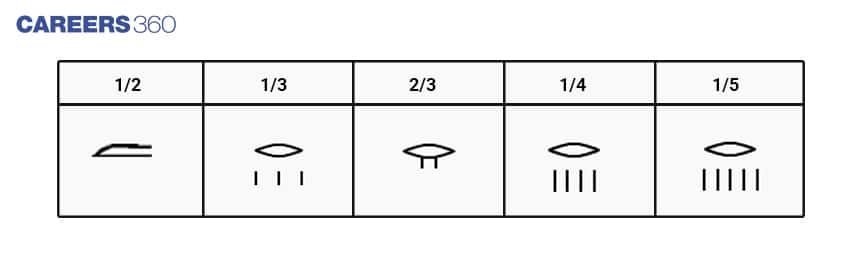Multiplication

Binary arithmetic was used for multiplication by Egyptians. The multiplication of two numbers was calculated by a repeated doubling of the multiplicand. Let's understand multiplication with an example.

Multiplication of 19 x 25

Series of binary numbers: 1, 2, 4, 8, 16, 32,...

 Binary numbers ( Power of 2) Multiplication of 25 1 25 2 50 4 100 8 200 16 400 32 800

19 can be written as the sum of 16 + 2 + 1

Therefore 19*25 = 16*25 + 2*25 + 25 = 400 + 50 + 25 = 475

Division

Binary arithmetics were also used for the division of numbers. A repeated halving technique is used. Let's understand with an example.

Division of 1075 / 25

 Binary numbers (Power of 2) Multiplication of 25 1 25 2 50 4 100 8 200 16 400 32 800

1075 can be written as 800 + 200 + 50 + 25

Therefore division 1075 / 25 = 32 + 8 + 2 + 1 = 43

## Algebra

Ancient Egyptian was the first civilization to use the second order quadratic equations. The information is found in different papyrus, which is plant-based writing material. Similar to the modern mathematical notation like alpha, beta, etc also found the papyrus was used to solve linear equations

## Geometry

The different papyrus shows the ancient Egyptians were aware of how to compute the area and volume of different shapes, for example, three dimensions cylinders and pyramids.

Area

Triangles: different papyrus shows that ancient Egyptians were known about computing the area of a triangle.

Rectangles: there are some problems in a papyrus regarding the area of a rectangular plot of land.

Circles: In a Rhind Mathematical Papyrus, there is a comparison between the area of a circle and its circumscribing square. This means they had an understanding of the area of a circle as well as the area of circumscribing squares.

Hemisphere: there is a problem in Mathematical papyrus in which calculation of the area of a hemisphere was discussed.

Volumes

Cylindrical (Cylinder): there are several problems in a papyrus of computation of the volume of cylindrical granaries, a pillar, or a cone instead of a pyramid.

Rectangular (Cuboid): there are several problems computing the volume of a rectangular granary

Truncated Pyramid (Frustum): In mathematical papyrus, The volume of a truncated pyramid is computed.

Seqed

Egyptians also had an understanding of geometric similarities. For describing the inclination of the triangle face they used the term “Seqed” which is a ratio of run/rise. This ratio is very important in the building of pyramids.

## Why Is It Relevant Today?

The historical developments in Mathematics are given in NCERT Class 9 chapter 5, “Introduction To Euclid’s Geometry”. In NCERT class 10 chapter 6 “triangle” students study the right angle triangle as well as the concepts of similar triangles. This article can help students to understand this historical development in Euclidean geometry. The following concepts of Mathematics developed and used by ancient Egyptians are still relevant.

Egyptian Triangle And Golden Ratio

The Egyptian triangle is a specific type of triangle with three sides, the 3-4-5 right angle triangle. The triangle name is driven by the functions in the construction of stonework and monuments. the mathematical principles that Egyptian builders developed and different geometrical shapes are still used.

The golden ratio is (1 + √5) / 2 which is approximately equal to 1.618. It is a ratio of a/b = (a+b)/a.

In the following image, φ shows the golden ratio.

### Egyptian Triangle And Golden Ratio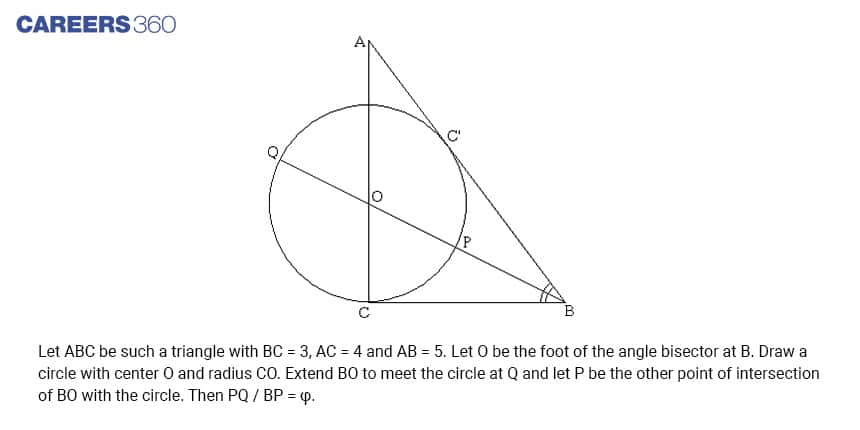Binary System

The binary system was used by Egyptians for multiplication. This concept of multiplication is still used as the base of modern computing. The binary system has only two levels namely 0 and 1.

Decimal Systems

Ancient Egyptians also made extensive use of the concept of decimal systems with no symbol for zero. This decimal system helps them to calculate quantities. At present time the decimal system is very useful for money, lengths, weight, etc. they use hieroglyphics for representation of the decimal system.

Fractions

Egyptians used fractions to calculate quantities. Fractions are very important at present time for the calculation of different quantities. Unlike today's number system Egyptians used a number system based on whole numbers

Hope this article helps you in understanding major concepts in ancient Egyptian Mathematics as well as why these are relevant today.

• Geometry
• Mathematics
• Mathematical objects
• Elementary mathematics
• Number
• Area
• Triangle
• Ratio
• Multiplication
• Decimal

## Careers360 helping shape your Career for a better tomorrow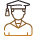#### 250M+

Students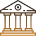#### 30,000+

Colleges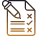#### 500+

Exams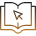E-Books